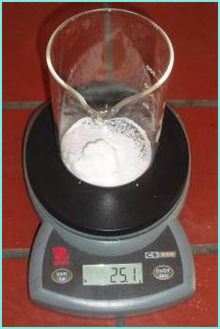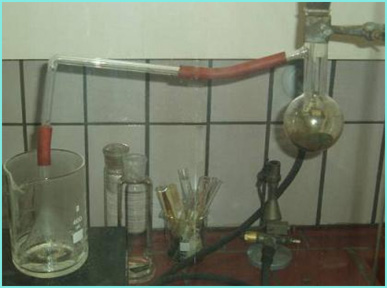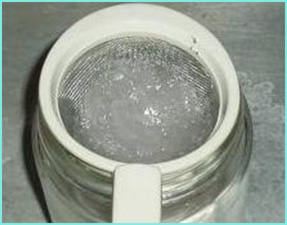Synthesis database: Potassium chlorate synthesis (via chlorine disproportionation) This method of potassium chlorate production is somewhat more complicated and dangerous than the hypochlorite method, mainly because one of the reagents needed here, is chlorine. Chlorine is, as most already know, a very poisonous gas. Because of that, one must be especially careful when doing this reaction. The reaction is based on the following equation: 3K2CO3 (aq) + 3Cl2 (g) -› 5KCl (aq) + KClO3 (aq) + 3CO2 (g) As it is mentioned in the title, this is basically a reaction of disproportionation of chlorine, because the oxidation number of chlorine is being both, reduced and increased during this reaction, from 0 (Cl2), to -1 (KCl) and +5 (KClO3).As one can see, potassium carbonate is one of the reagents, and it must be dissolved in distilled water. The second reagent is chlorine which is produced in a reaction of potassium permanganate and concentrated hydrochloric acid. The reaction can be represented by the following equation: 2KMnO4 (s) + 16HCl (aq, konc.) -› 5Cl2 (g) + 2KCl (aq) + 2MnCl2 (aq) + 8H2O (l) First, 25 grams of potassium carbonate (0.180 moles) are taken and added to 17.5 mL of distilled water. This solution is then gradually heated in a glass beaker. The solution is stirred from time to time, to speed up the dissolving of the K2CO3. In the meanwhile, the apparatus for the generation of chlorine is prepared. Basically, one needs a reaction beaker in which the hydrochloric acid and potassium permanganate will react, a funnel with a stopper (in which one will pour the HCl solution), and an exit hose which will lead to a beaker containing the K2CO3 solution. Before setting the apparatus, 11.4 grams (0.072 moles) of potassium permanganate is added to the reaction beaker. To the funnel, 21.1 grams (0.576 moles) of concentrated hydrochloric acid is added. The mentioned amounts of compounds should yield about 0.180 moles of molecules of chlorine, exactly the amount which is needed for the reaction with 0.180 moles of potassium carbonate.After the apparatus is ready, one should wait with the reaction until the solution of potassium carbonate reaches its boiling point. After that, one can begin to add the hydrochloric acid, drop by drop, to the potassium permanganate. Chlorine starts to form immidiately and flows through the hose into the solution of potassium carbonate. The chlorine is slowly generated through a longer period of time (little more than 1/2 h). At the end of the reaction, some crystals may be visible on the walls of the beaker with the K2CO3 solution. This is due to the large amount of KCl that is produced together with the KClO3. After the reaction is completed, one can simply add a bit more distilled water to the still hot solution, to dissolve the remaining crystals. However, one shouldn't add too much water, as the next step is to pour the solution in a wider beaker, so that water could evaporate at a relatively fast rate. After drying, the result is a mixture of solid potassium chlorate and potassium chloride. In my example, I got about 28.8 grams of this mixture. Having in mind the amounts of the reagents in the chemical equation, theoretical mass of KCl should have been around 22.3 grams, and the theoretical mass of KClO3 should have been around 7.3 grams. So the sum of these two equals 29.6, and as one can see, the experimental yield is very close to that value. In order to separate these two compounds, one should add about 80 mL of cold (0 °C) distilled water to the mixture of salts. The solubility of KCl is much higher than that of KClO3, so that after some stirring, one can easily filter out the relatively pure crystals of KClO3, while the KCl stays in the solution. Unfortunately, some of the KClO3 will dissolve in the water, but the loss is negligible.After the crystals of KClO3 have been filtered, they can be added to a small container and placed in a desiccator (containing, for example, silica gel) in order to dry the crystals properly.